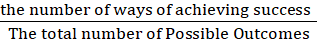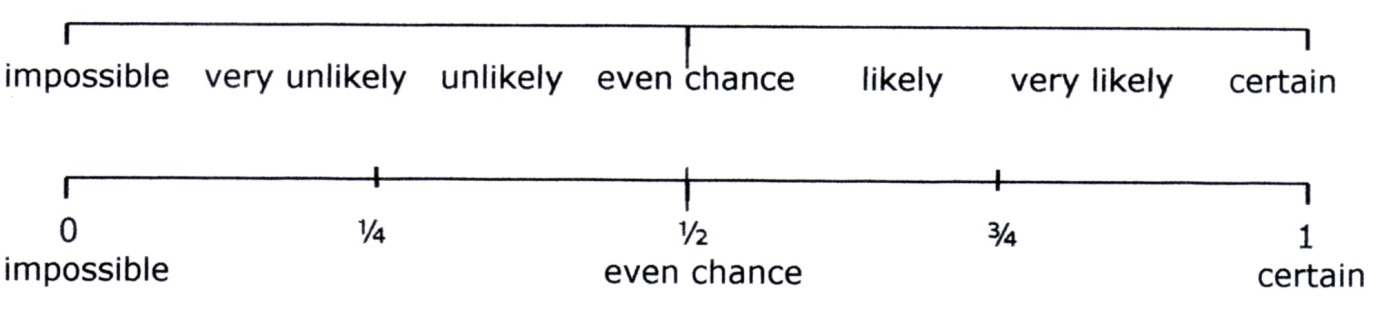# RD Sharma Class 11 and Chapter Probability Maths Solutions

## Maths Class 11 and Chapter Probability RD Sharma SolutionsFilter
Filters :
CLASSES
•
•
•
•
•
•
•
CHAPTERS
•
• 23 More

### RD SHARMA Class 11 | PROBABILITY

A bag contains 8 red, 3 white and 9 blue balls. If three balls are drawn at random, determine the probability that (i) all three balls are blue balls (ii) all the balls are of different colours.### RD SHARMA Class 11 | PROBABILITY

The face cards are moved from a full pack. Out of the remaining 40 cards, 4 are drawn at random. What is the probability that they belong to different suits?RD Sharma Chapter 33, Probability, is a very important topic in the Mathematics syllabus of Class 11th. Although, this chapter may seem like quite an easy-breezy to learn, however, the questions asked in the examination can be tricky from this this chapter. Therefore, it is important for students to master the given RD Sharma Class 11 Solutions - Chapter 33, Probability, in order to remain competitive in board and engineering entrance examination. To gain a competitive edge in this chapter, it is essential for you to have a strong foundation in the concepts of probability. Therefore, the solutions provided here by Doubtnut experts are prepared keeping in mind to help students learn this chapter from very basics. In this RD Sharma Chapter 33 we will be studying the methods of calculating the probability of an incident, be it intricate or simple. You’ll be introduced to various types of tools used in the probability calculations.  We’ve also provided sufficient number of examples and previous year solved questions to make it easily comprehensible and clearly understandable for you.

Listed below are important topics and sub-topics of RD Sharma Class 11 solutions of Chapter 33 Probability:

1. Introduction and Definition of Probability
2. Probability Line
3. Probability vs. Statistics
4. Experiment or Trial
5. Sample Space and Sample Point
6. Event: Types of Events, Complement of an Event, Mutually Exclusive Events (Disjoint Events), Independent Events
7. Probability Distribution
8. Conditional Probability
9. Bayes Theorem
10. Binomial Distribution for Successive Events
11. Assumptions of Binomial Distribution

In RD Sharma Class 11 Chapter 33 Probability, you will find several solved and unsolved exercise questions. We have provided complete RD Sharma solution for Probability to help the students and understand the concepts better. You can check the solutions for the exercises given in RD Sharma book to learn about it in detail. The solutions are in a step by step manner and are made available in exercise wise pattern.

## Topics and Subtopics of RD Sharma Class 11 Solutions Chapter 33 Probability

### What is Probability?

In simple words, Probability is the likelihood of an even to occur. It is the degree of likeliness of the happening of an event. Probabilities are given a value between 0 and 1. Zero percent chance implies that an even will not happen and a 100% chance implies the best possibility of an even to happen. The higher the degree of probability, the more likely the event is to happen and vice versa.

Probability = the number of ways of achieving success  The total number of Possible Outcomes### The probability of tossing a coin

When we toss a coin there are just two possible outcomes i.e. either H (heads) or T (Tails). As the coin is always unbiased the probability of both the possible outcomes are equal i.e. half. We write P (heads) =1/2 and P (Tails) = 1/2.

Note that the Probability is a guide, it never tells us exact outcome, and rather it gives possible expectancy of an event to happen. Suppose we toss a coin hundred times, so many times do you think the heads will come up? You may think 50 heads, as per the above example, the probability that head turns up are half. But when we actually try it, the possible outcomes we get might be 41, 49, 51 or 59 or any number near to 50, but not precisely 50.

### Throwing a dice

As we all know that a dice is a well-balanced cube. It has six marked faces: 1, 2, 3, 4, 5, 6, i.e. one number on each face. When a dice is thrown there can be 6 possible outcomes as any number among 1 to 6 can appear on the upper face. Since there are six equally likely outcomes therefore, we write this as

P (1, 2, 3, 4, 5, 6) = 1/6

Since it is not possible to get a 7 or any other number on a dice hence, its probability is zero.

### The Probability LineProbability is always between 0 and 1.

General Outcomes of Probability:

1. The probability of something which is definite to happen = 1
2. The probability of something which is impossible to happen = 0
3. The probability of something not happening is = - 1 minus the probability that it will happen

### Probability and Statistics

Probability and statistics are two related but distinct academic fields. Statistics often utilizes probability, and the two mathematical topics are often learnt together. In the both branches of mathematics, you will be studying the possible outcomes of events and examination of random occurrences, analysis and demonstration of mathematical data.

## RD Sharma Class 11 Solutions Chapter 33 Probability

Get familiar with the important concepts of RD Sharma Class 11 Solutions – Chapter 33, Probability, with the help of free video tutorials by doubtnut. The RD Sharma Class 11 Solutions - Chapter 33 Probability comprising study notes, video lectures, and previous year solved questions, etc. These 5-10 minutes of pre-recorded RD Sharma tutorials are meant for your thorough understanding of each & every topic. Open any Class 11 Maths Solutions video and you will find an expert guiding your way. With the convenience of learning in HD prime quality. Simply take notes from these tutorials religiously to score best grades in your CBSE board examination. Also, you can anytime rewind or fast-forward the videos as per your comfort and pace of learning.

## Get Started with Doubtnut.com

At Doubtnut, we strive to facilitate the easy and rightful access to quality study materials to students of all age groups. You can also download our App “Doubtnut” from Google play store. With the highest 4.4 play store rating, Doubtnut App is the student’s favorite e-learning destination to prepare for competitive and board examination. Keep visiting doubtnut.com for more interactive study material!